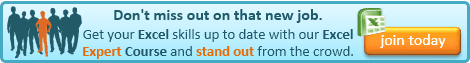## Tuesday, 9 September 2014

### Excel Tip: SUBTOTAL and SUM - what's the difference?

I'm sure most of you are familiar with the SUM command for totalling ranges of cells, but have you heard of the SUBTOTAL command? - and if so, do you know what it does?

I must admit that I had only looked at the SUBTOTAL command in any detail this week - prompted by a question from a reader. I had never really used it, because I normally advocate doing any analysis of a data list separately using PivotTables, rather than within the list itself and didn't realise that it offered some interesting differences to just using the SUM command.

At first glance it would appear to be the same, but it has a number of additional powers!

I am aware that most of you will know this next bit, but I have included it for completeness:

The SUM command is used as follows:

=SUM(number1,[number2],....)

only number1 is required and should be a number, or a reference to a number or range of numbers.

So, number1 could be, for example, 3, A4, A1:A4 or a named range.

The same rules apply to number2 and number3 all the way up to number255, except these are all optional.

The SUM function then returns the total of all of these numbers.

The SUBTOTAL command has very similar arguments except it has one additional argument in front of them:

=SUBTOTAL(function_num,ref1,[ref2],....)

ref1 and the optional arguments ref2 to ref254 are very similar to number1, etc. in the SUM function, however they must be references to cells or ranges of cells - i.e. not actual numbers.

The function_num argument must be between 1 and 11 or between 101 and 111. This argument determines how the function is to summarise the numbers. The Excel function that will be applied for each of function_num 1 to 11 is given below:

1 AVERAGE
2 COUNT
3 COUNTA
4 MAX
5 MIN
6 PRODUCT
7 STDEV
8 STDEVP
9 SUM
10 VAR
11 VARP

This is the first real difference between SUM and SUBTOTAL. SUBTOTAL can mimic any of these 11 functions.

The second difference is more subtle. You would expect the following two functions to return the same result:

=SUM(A1:10)
=SUBTOTAL(9,A1:10)

and in most cases they will. The difference becomes apparent when you apply a filter to the data. This will have no effect on the SUM result but the SUBTOTAL will exclude any rows hidden by the filter from the calculation. This could be very useful if you regularly work with a data table that you filter in place using the AutoFilter facility, as it will show you the total of the displayed rows.

You can further affect the result by using function_num 101 to 111. These work exactly the same as 1 to 11 but exclude rows hidden using the Hide command as well.

One final difference is that the SUBTOTAL command will ignore any other SUBTOTAL commands in the range being summed, thereby avoiding double-counting. Thanks to Jeremy for pointing out that I'd missed that one!If you enjoyed this post, go to the top of the blog, where you can subscribe for regular updates and get two freebies "The 5 Excel features that you NEED to know" and "30 Chants for Better Charts".

1.the biggest difference I find is that if you work with subtotals, and use SUM for each subtotal, then at the bottom, you will have to select each subtotal cell individually. Otherwise, using SUM on the whole column will include all values and their subtotals. However using SUBTOTAL avoids this problem, SUBTOTAL (9,...) will not include other subtotals in the result.

1.Thanks for that Jeremy. To be honest I'd meant to include that and completely forgot when I wrote the article! I'll put an amendment at the bottom now.

2.Really useful stuff, Glen

I use Subtotal 109 a lot in the Total Row of Data Tables.

And I have found the following tip/trick works well -
If you copy and paste the Subtotal Formula from one Column total cell to another, the name of the original column remains in the Structured Reference. However, if you drag the formula between the two total cells using the drag handle at the bottom right of the cell, it automatically inserts the name of the other column for you. Cool!

1.Thanks Dave, that's a handy tip!